## 11-3 ODE 嚙踝蕭嚙踝蕭嚙踝蕭嚙踝蕭嚙踝蕭嚙踝蕭嚙踝蕭嚙?

[t, y] = solver('odeFile', [t0, tn], y0, options);

options = odeset('name1', value1, 'name2', value2, …)

newOptions = odeset(options, 'name', value);

value = odeget(otpions, 'name');

Example 1: 11-常微分方程式/odeset01.modeset AbsTol: [ positive scalar or vector {1e-6} ] RelTol: [ positive scalar {1e-3} ] NormControl: [ on | {off} ] NonNegative: [ vector of integers ] OutputFcn: [ function_handle ] OutputSel: [ vector of integers ] Refine: [ positive integer ] Stats: [ on | {off} ] InitialStep: [ positive scalar ] MaxStep: [ positive scalar ] BDF: [ on | {off} ] MaxOrder: [ 1 | 2 | 3 | 4 | {5} ] Jacobian: [ matrix | function_handle ] JPattern: [ sparse matrix ] Vectorized: [ on | {off} ] Mass: [ matrix | function_handle ] MStateDependence: [ none | {weak} | strong ] MvPattern: [ sparse matrix ] MassSingular: [ yes | no | {maybe} ] InitialSlope: [ vector ] Events: [ function_handle ]

AbsTo1正純量或向量$10^{-6}$絕對誤差容忍度

OutputSel索引向量全部ODE 指令之輸出變數的索引值，以決定那些輸出變數之元素將被送到輸出函式
Refine正整數1或4 (for ode45)Refine = 2 可產生兩倍數量的輸出點，Refine = 3 可產生三倍數量的輸出點，依此類推。
Statson 或 offoffStats = 'on' 產生計算過程的各種統計資料。
Jacobian 矩陣之相關欄位Jconstanton 或 offoff如果 Jacobian 矩陣常數，則 Jconstant = 'on'
Jacobianon 或 offoff若F(t, y, 'Jacobian') 傳回 $\partial F/\partial y$，則 Jacobian = 'on'
Jpatternon 或 offoff若 F([ ], [ ], 'JPattern') 傳回 $\partial F/\partial y$，且 $\partial F/\partial y$ 是稀疏矩陣，則 Jpattem = 'on'
Vectorizedon 或 offoff若 F(t, [y1, y2…..]) 傳回 [F(t,y1), F(t,y2)…..]，則 Vectorized = 'on'

Initial Step正純量 起始步長的建議值

MassSingularyes，no 或 maybemaybe表明質量矩陣是否為 Singular

Ode15s 之相關欄位MaxOrder1, 2, 3, 4 或 55積分公式用到的最高次數
BDFon 或 offoff若使用 BDF（Backward Differentiation Formula）則 BDF = 'on'

f 在積分誤差容忍度方面，每一次積分所產生的局部誤差 e(i)，必須滿足下列方程式：

$$|e(i)| \leq max(RelTol*|y(i)|, AbsTol(i))$$

Example 2: 11-常微分方程式/odeRelTol01.msubplot(2,1,1); ode45('vdp1', [0 25], [3 3]'); title('RelTol=0.01'); options = odeset('RelTol', 0.00001); subplot(2,1,2); ode45('vdp1', [0 25], [3 3]', options); title('RelTol=0.0001');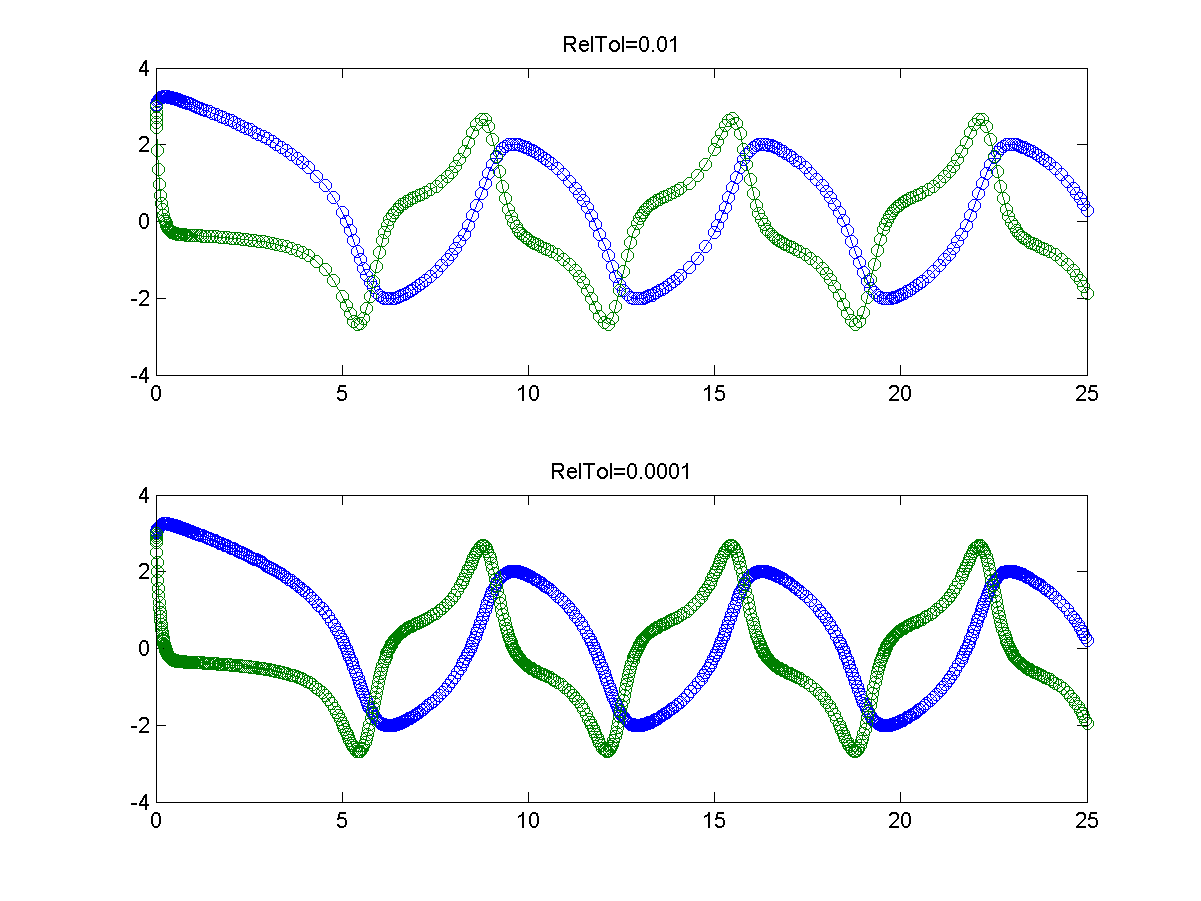• odephas2：畫出 2-D 的相位平面（Phase Plane）
• odephas3：畫出 3-D 的相位平面
• odeprint：隨時在指令視窗印出計算結果

11-常微分方程式/lorenzOde.mfunction dy = lorenzOde(t, y) % LORENZODE: ODE file for Lorenz chaotic equation SIGMA = 10.; RHO = 28; BETA = 8./3.; A = [ -BETA 0 y(2) 0 -SIGMA SIGMA -y(2) RHO -1 ]; dy = A*y;

Example 3: 11-常微分方程式/odeLorenz01.mode45('lorenzOde', [0 10], [20 5 -5]');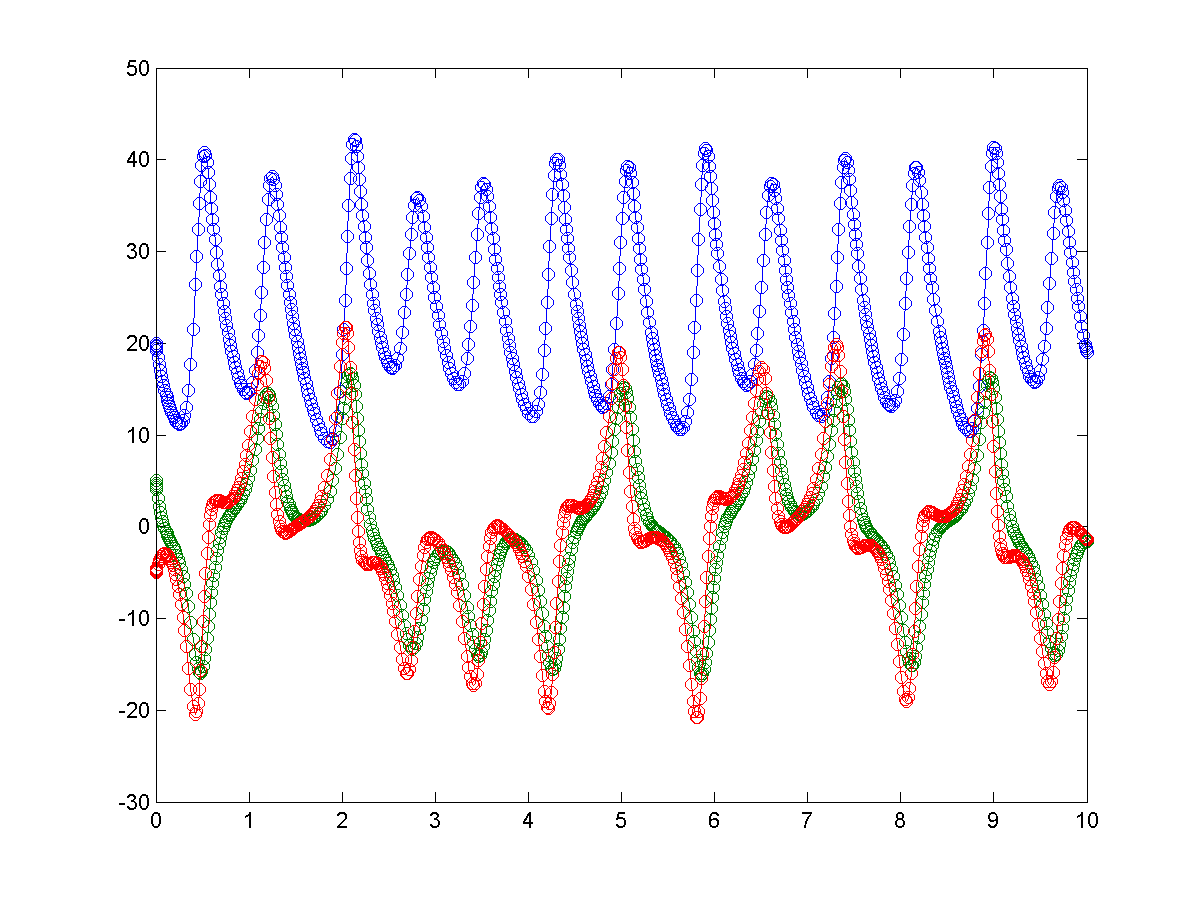Example 4: 11-常微分方程式/odeLorenz02.moptions = odeset('OutputFcn', 'odephas3'); % 使用 odephas3 進行繪圖 ode45('lorenzOde', [0 10], [20 5 -5]', options);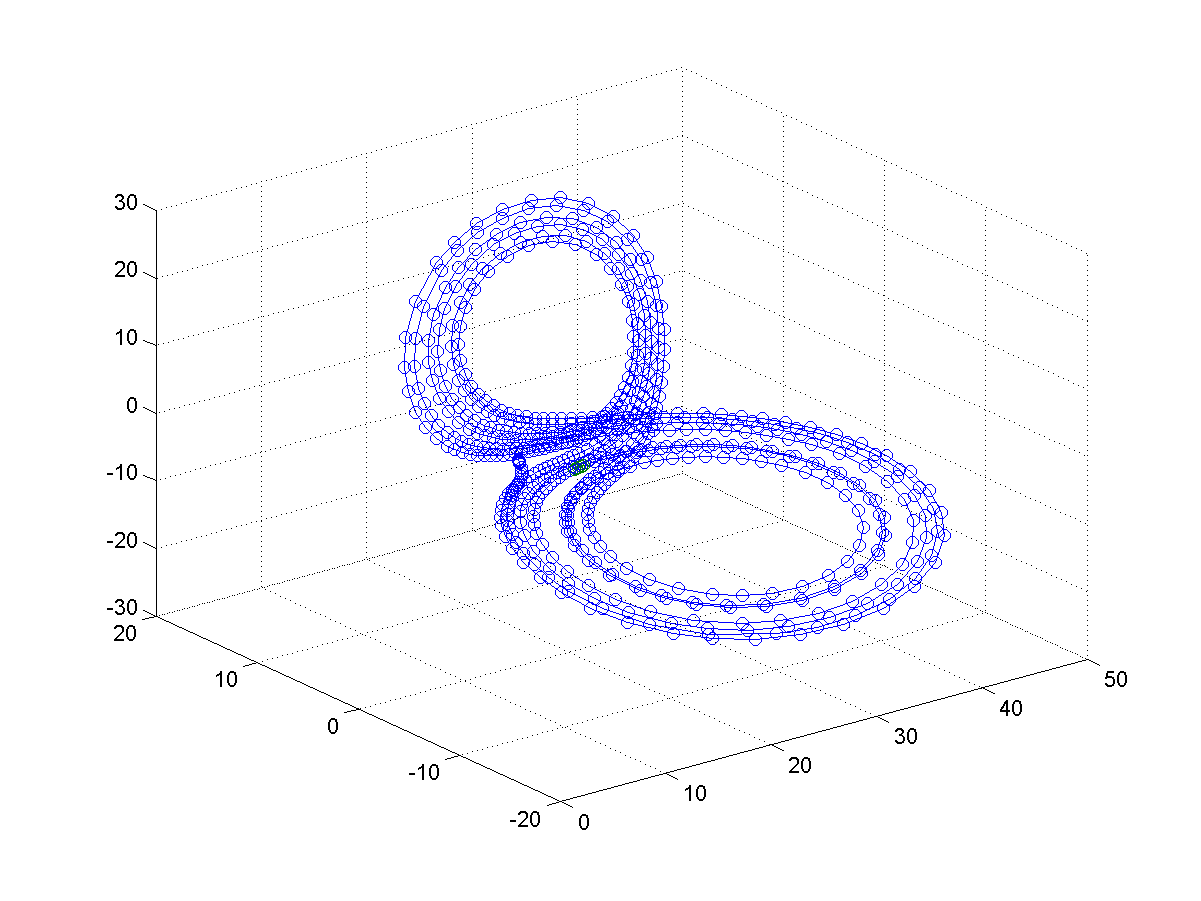Hint

options = odeset('OutputFcn', 'myFunc')

1. 積分開始時，ODE 指令會呼叫 myFunc(tspan, y0, 'init') 以讓 myFunc 進行各種初始化動作。
2. 在每個積分步驟中，ODE 指令將會持續呼叫 status=myFunc(t, y)，若 status=1，則停止積分。
3. 在積分結束時，ODE 指令會呼叫 myFunc([], [], 'done')，以讓 myFunc 進行收尾動作。

OutputSel 可指定要傳送到 OutputFcn 的狀態變數之元素。例如，若只要傳送第一及第三個 Lorenz 渾沌方程式的狀態戀數至 odeplot，可輸入如下：

Example 5: 11-常微分方程式/odeOutputSelect01.moptions = odeset('OutputSel', [1,3]); % 只畫出第一和第三個狀態變數 ode45('lorenzOde', [0 10], [20 5 -5]', options);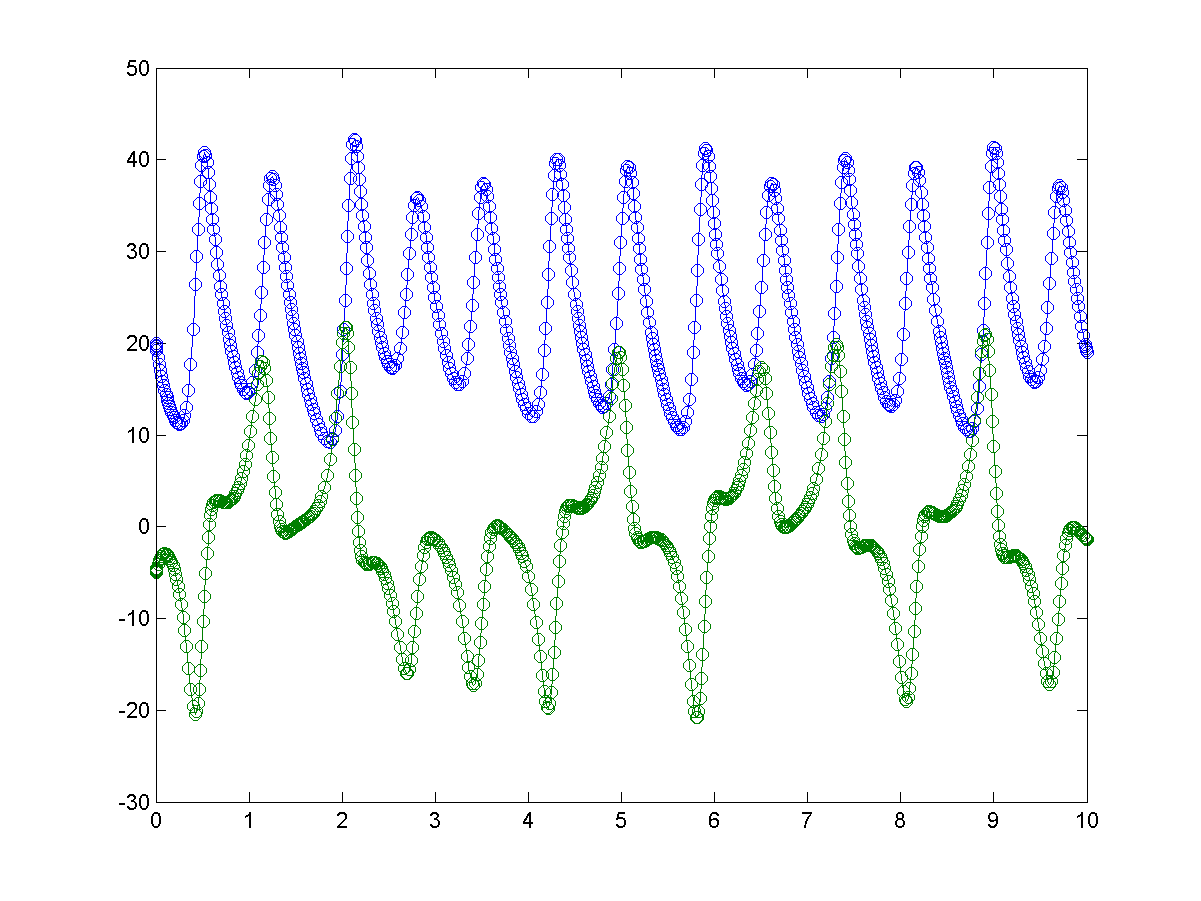Refine 欄位可以使用內插法來增加輸出狀態變數的密度，以得到較平滑的輸出曲線。在下例中，我們利用 Refine 欄位來使 ode23 的輸出點個數增為原先的三倍：

Example 6: 11-常微分方程式/odeRefine01.msubplot(2,1,1); ode23('vdp1', [0 25], [3 3]'); subplot(2,1,2); options = odeset('Refine', 3); ode23('vdp1', [0 25], [3 3]', options);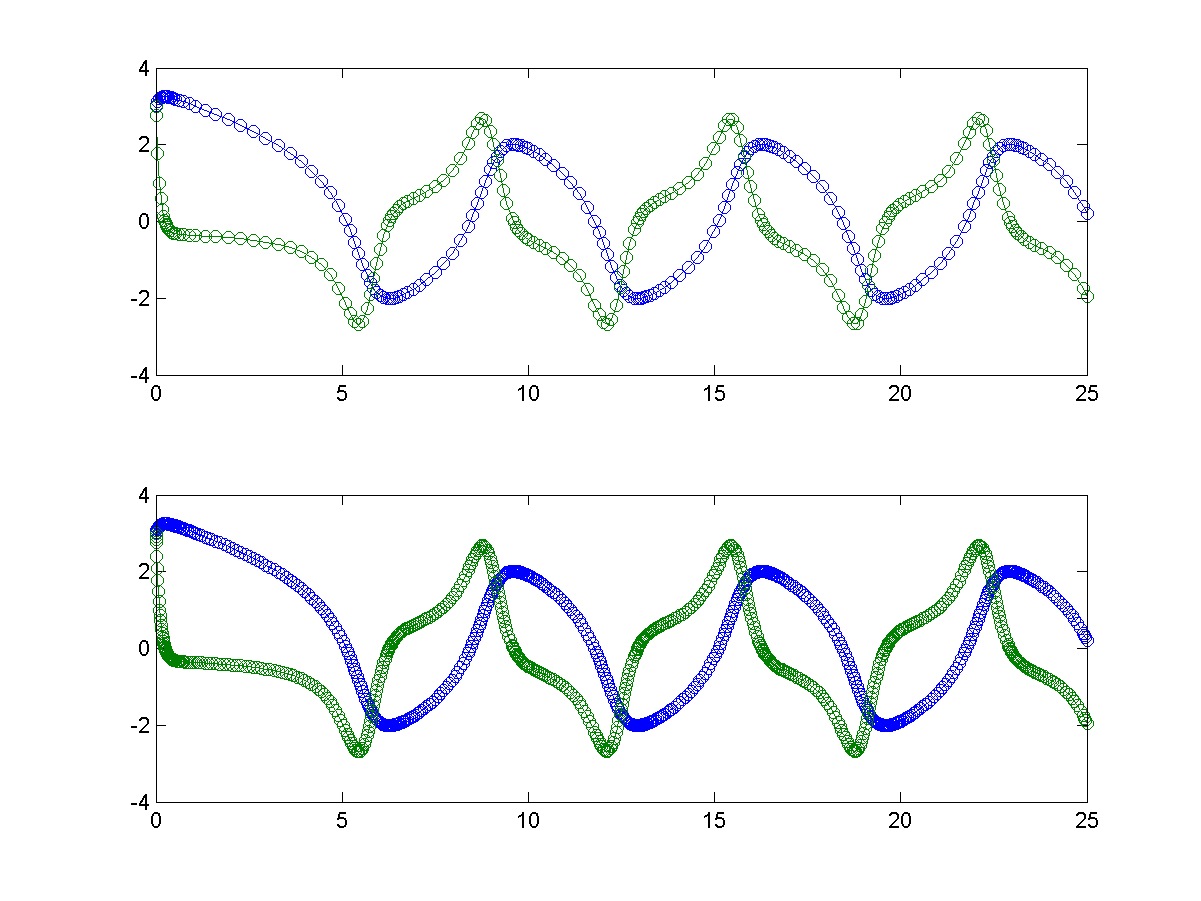Example 7: 11-常微分方程式/odeShowStats01.m[t, y] = ode45('vdp1', [0 25], [3 3]', odeset('Stat', 'on'));71 successful steps 10 failed attempts 487 function evaluations

Example 8: 11-常微分方程式/odeShowStats02.m[t, y, s] = ode45('vdp1', [0 25], [3 3]'); ss = 71 10 487 0 0 0

MaxStep 及 InitialStep 欄位可用來調整最大積分步長及起始積分步長。但一般而言，您不必去調整這兩個數值，因為 ODE 指令本身就具有步長自動調適功能。特別值得注意的是：

• 若要產生更多輸出點，可直接調整 Refine 欄位值。調整 MaxStep 雖然可以達到同樣效果，但是計算時間可能會大幅增加。
• 如果積分結果不甚準確，請勿先調降 MaxStep，您應先調降 RelTol 及 AbsTol。調降 MaxStep 是最後的步驟。

MATLAB程式設計：進階篇